# Simple Square wave to Sine Wave Converter

Published  July 17, 2020   1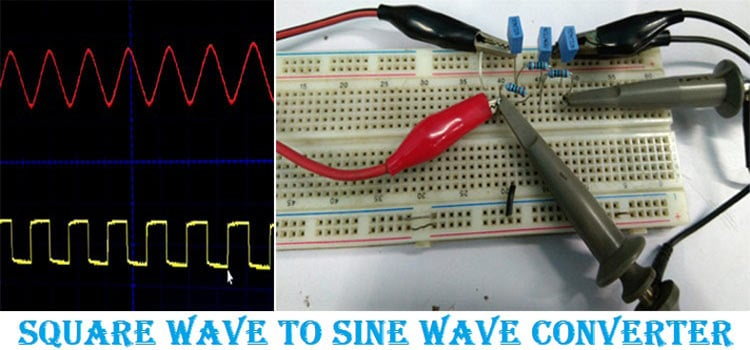Square wave to Sine wave converter circuit is an important analog circuit that converts square waveforms to sine waveforms. It has a broad spectrum of applications in many different areas of electronics, such as in mathematical operations, acoustics, audio application, inverters, power source, function generator, etc.

In this project, we will discuss how a square wave to sine wave converter circuit works and how it can be built using simple passive electronics. You can also check out other waveform generator circuits listed below.

### Square to Sine Wave Converter using RC Network

A square wave to sine wave converter can be built using 6 passive components, namely capacitors and three resistors. Using these three capacitors and three resistors, a 3 stage RC network can be built that takes a square wave as an input and sine wave as an output. A simple single stage RC network circuit is shown below.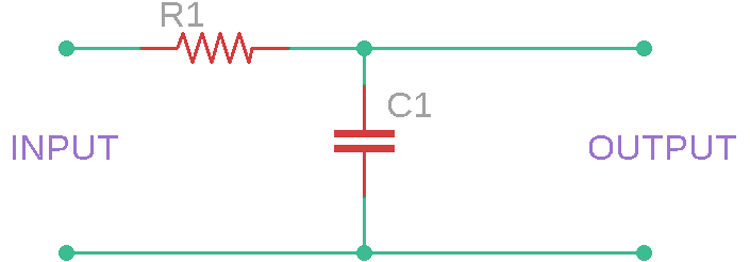In the above circuit, a single-stage RC filter is shown where a single resistor and a single capacitor is used. The above circuit is pretty simple. The capacitor gets charged depending on the status of the square wave. If the square wave in the input is in a high position, the capacitor will get charged, and if the square wave is in a low position, the capacitor gets discharged.

A varying signal wave such as a square wave has a frequency, depending on this frequency, the output of the circuits gets changed. Due to this behavior of the circuit, the RC filter is called an RC integrator circuit. An RC integrator circuit changes the signal output depending on the frequency and could change the square wave to a triangular wave or triangular wave to a sine wave.

### Square to Sine Wave Converter Circuit Diagram

In this tutorial, we are using these RC integrator circuits (RC filter networks) to convert square wave to sine wave. The complete converter circuit diagram is given below, and as you can see, it has only very few passive components.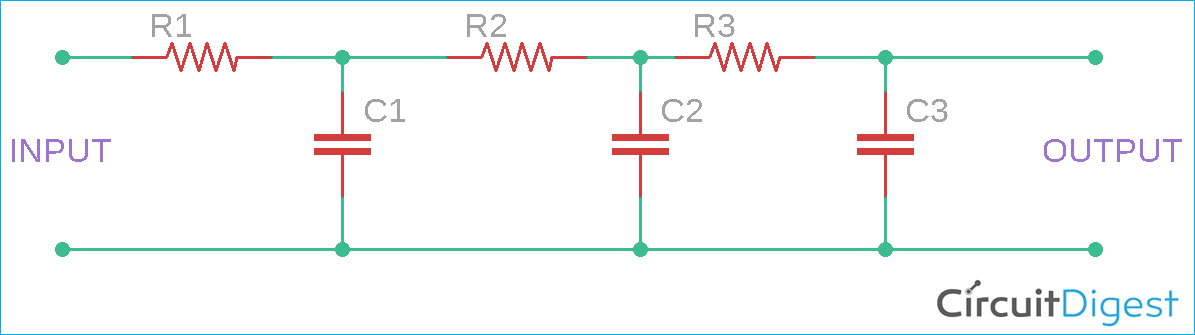The circuit consists of three stages of RC filter circuits. Each stage has its own conversion significance, let's understand the working of each stage and how it contributes to converting square wave to sine wave by looking at the waveform simulation

### Working Principle of Square Wave Converter

To know how the square wave to sine wave converter works, one needs to understand what is happening in each RC filter stage.

First Stage:

In the first RC network stage, it has a resistor in series and capacitor in parallel. The output is available across the capacitor. The capacitor gets charged up via the resistor in series. But, as the capacitor is a frequency-dependent component, it takes time to charge. However, this charge rate can be determined by the RC time constant of the filter. By the charging and discharging of the capacitor, and since the output comes from the capacitor, the waveform is highly dependent on the capacitor charge voltage. The Capacitor voltage during the charge time can be determined by the below formula-

`VC = V (1 – e-(t/RC))`

And discharge voltage can be determined by–

`VC = V (e-(t/RC))`

Therefore, from the above two formulas, the RC time constant is an important factor to determine how much charge the capacitor stores as well as how much discharging is done for the capacitor during an RC time constant. If we select the value of the capacitor as 0.1uF and the resistor as 100 k-ohms like the below image, it will have a time constant of 10 mili-seconds.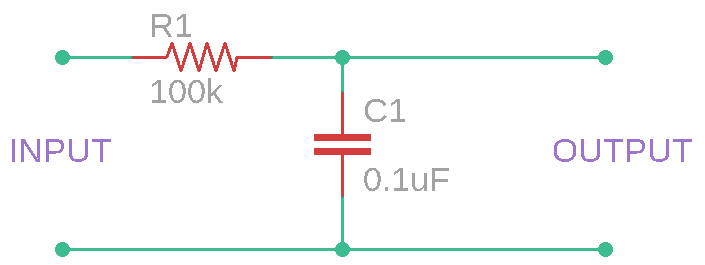Now, if a 10ms of a constant square wave is provided across this RC filter, the output waveform will be like this due to the charging and discharging of the capacitor in the RC time constant of 10ms.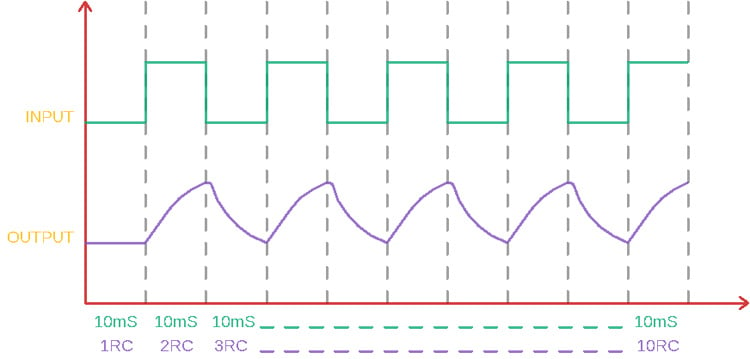The wave is the parabolic-shaped exponential waveform.

Second Stage:

Now the output of the first RC network stage is the input of the second RC network stage. This RC network takes the parabolic-shaped exponential waveform and makes it a triangular waveform. By using the same RC constant charging and discharging scenario, the second stage RC filters provide a straight ascending slope when the capacitor gets charged and a straight descending slope when the capacitor gets discharged.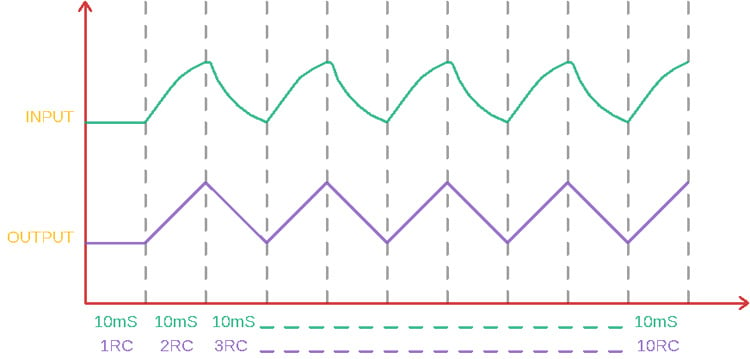The output of this stage is ramp output, a proper triangular wave.

Third Stage:

In this third RC network stage, the output of the second RC network is the input of the third RC network stage. It takes the triangular ramp wave as an input and then changes the shapes of the triangular waves. It provides a sine wave where the upper and lower portion of the triangular wave smoothen out making them curved. The output is pretty close to a sine wave output.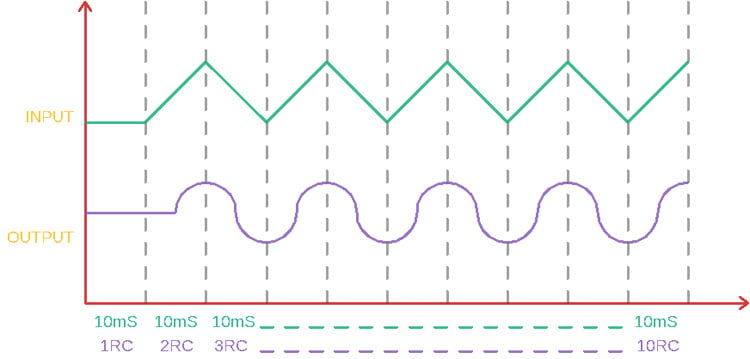### Selecting R and C values for Square Wave Converter Circuit

The capacitor and resistor value is the most important parameter of this circuit. Because, without the proper capacitor and resistor value, the RC time constant will not be matched for a particular frequency and the capacitor will not get enough time to charge or discharge. This results in a distorted output or even at high frequency, the resistor will work as an only resistor and could produce the same waveform as it was given across the input. So, capacitor and resistor values must be chosen properly.

If the input frequency can be changed, then one can choose a random capacitor and resistor value and change the frequency according to the combination. It is good to use the same capacitor and resistor value for all filter stages.

For a quick reference, at low frequencies, use a higher value capacitor, and for high frequencies, choose a lower value capacitor. However, if all the components, R1, R2, and R3 are the same value and all capacitors C1, C2, C3 are the same value, the capacitor and resistor can be selected using the below formula–

`f = 1/(2π x R x C)`

Where F is the frequency, R is the resistance value in Ohms, C is the capacitance in Farad.

Below schematic is a three-stage RC integrator circuit that is described previously. However, the circuit uses 4.7nF capacitors and 1 kilo-ohms resistors. This creates an acceptable Frequency range in the 33 kHz range.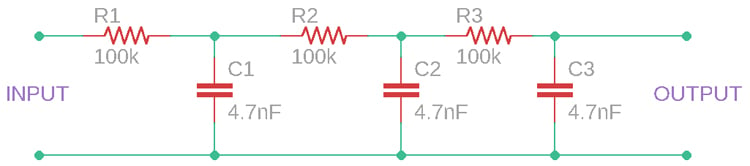### Testing our Square to Sine Wave Converter Circuit

The schematic is made in a breadboard and a function generator along with an oscilloscope is used to check the output wave. If you do not have a Function generator to generate the square wave, you can either build your own square wave generator or even an Arduino Waveform Generator which you can use for all waveform related projects. The circuit is very simple and hence it is easily built on the breadboard as you can see below.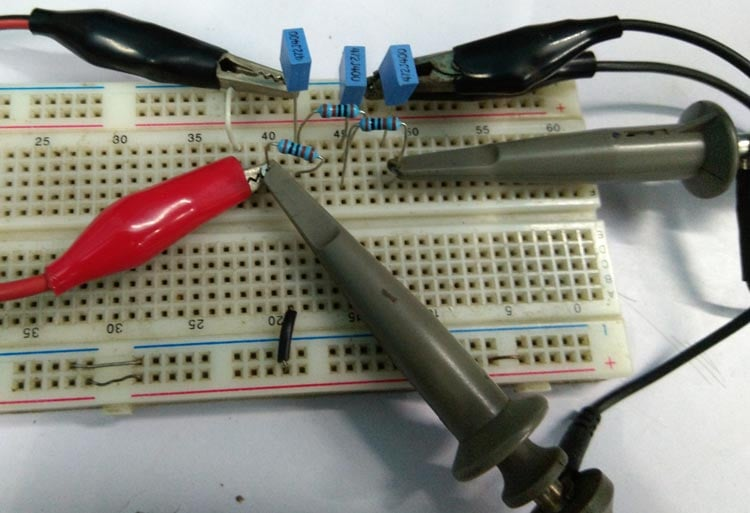For this demonstration, we are using a function generator and as you can see in the below image, the function generator is set to the desired 33 kHz square wave output.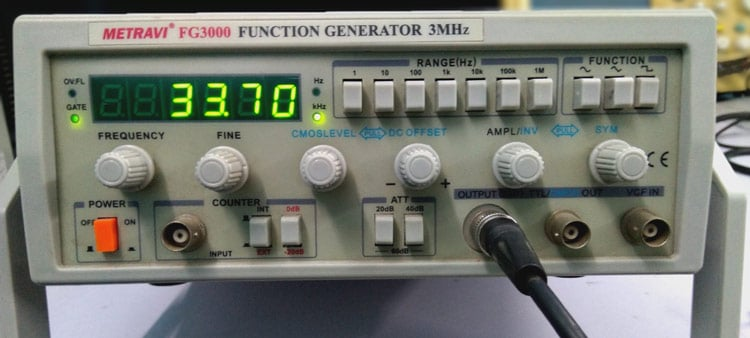The output can be observed on an oscilloscope, a snapshot of the output from the scope is given below. The input square wave is shown in yellow color and the output sine wave is shown in red color.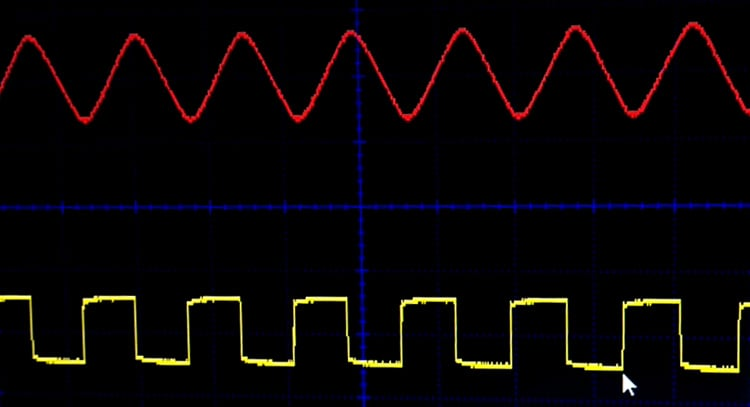The circuit worked as expected for an input frequency ranging from 20kHz to 40kHz, you can refer the video below for more details on how the circuit works. Hope you enjoyed the tutorial and learned something useful. If you have any questions, leave them in the comment section below. Or you can also use our forums to post other technical questions.

Video